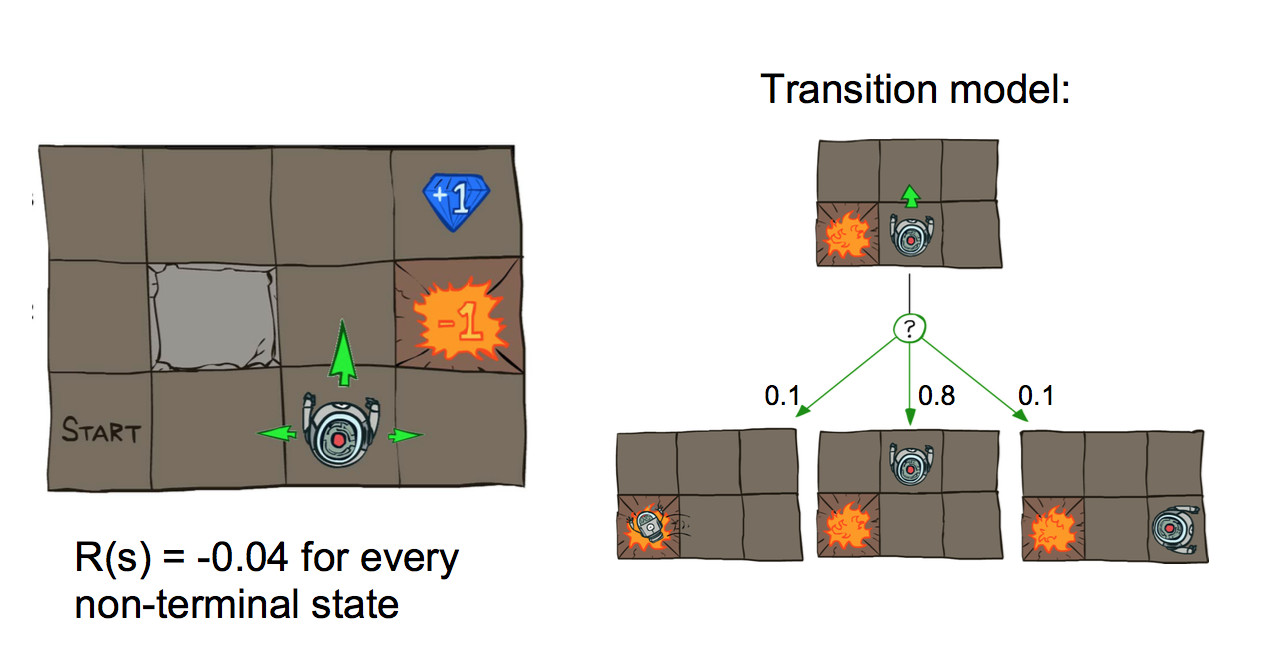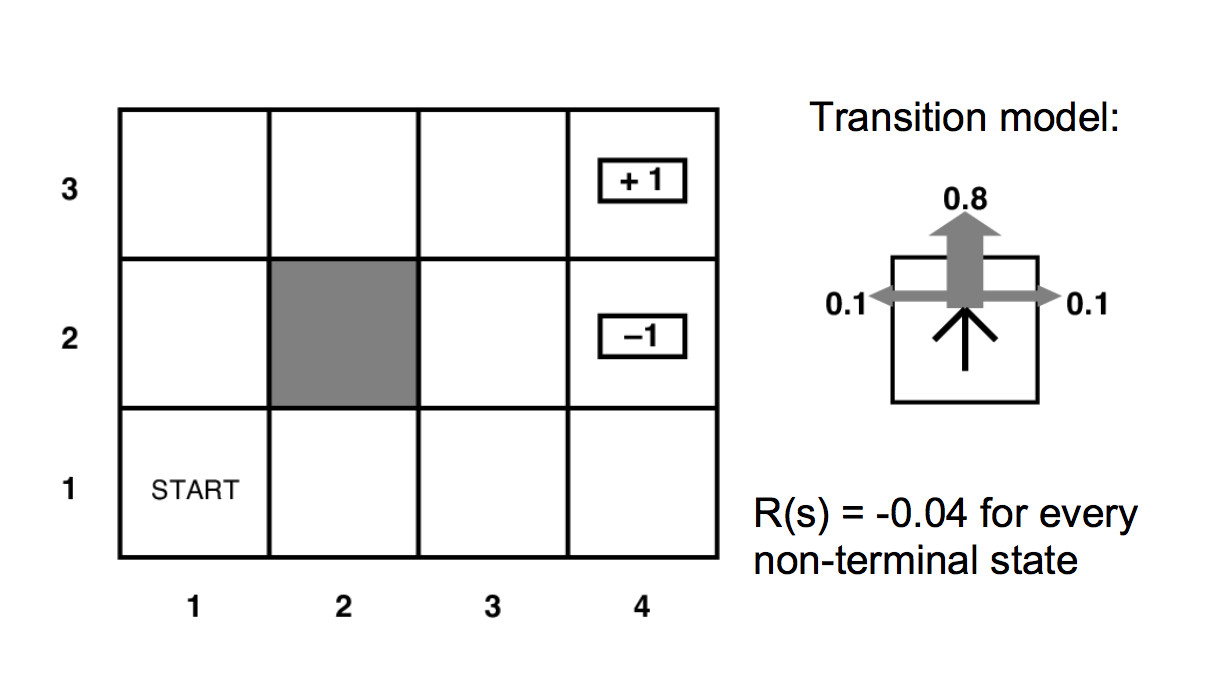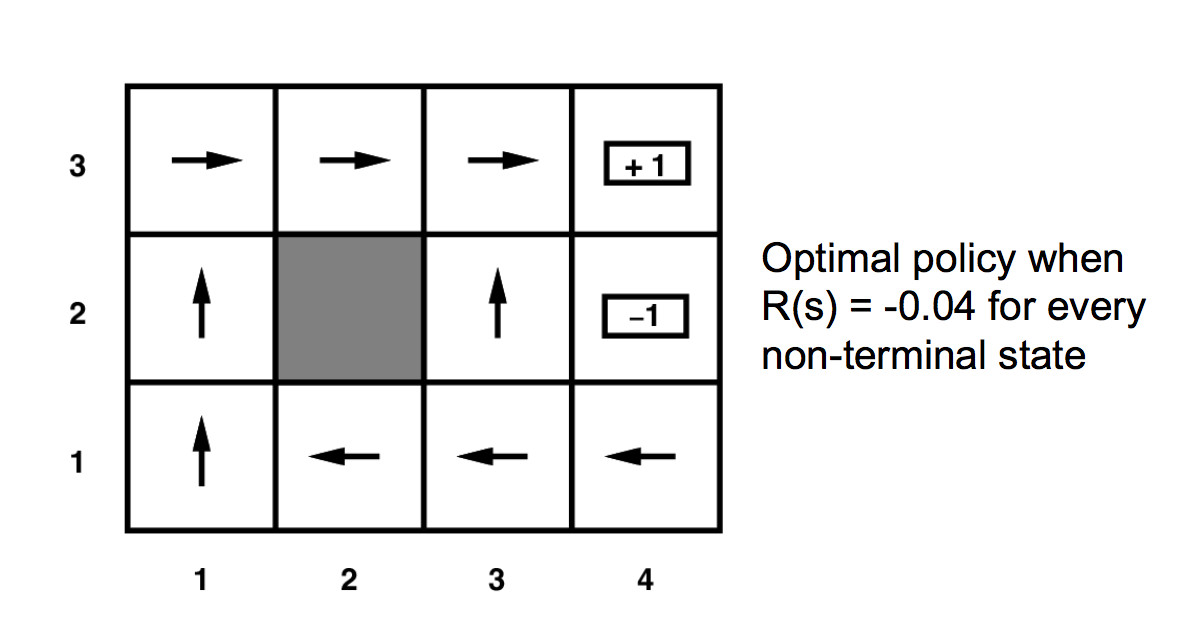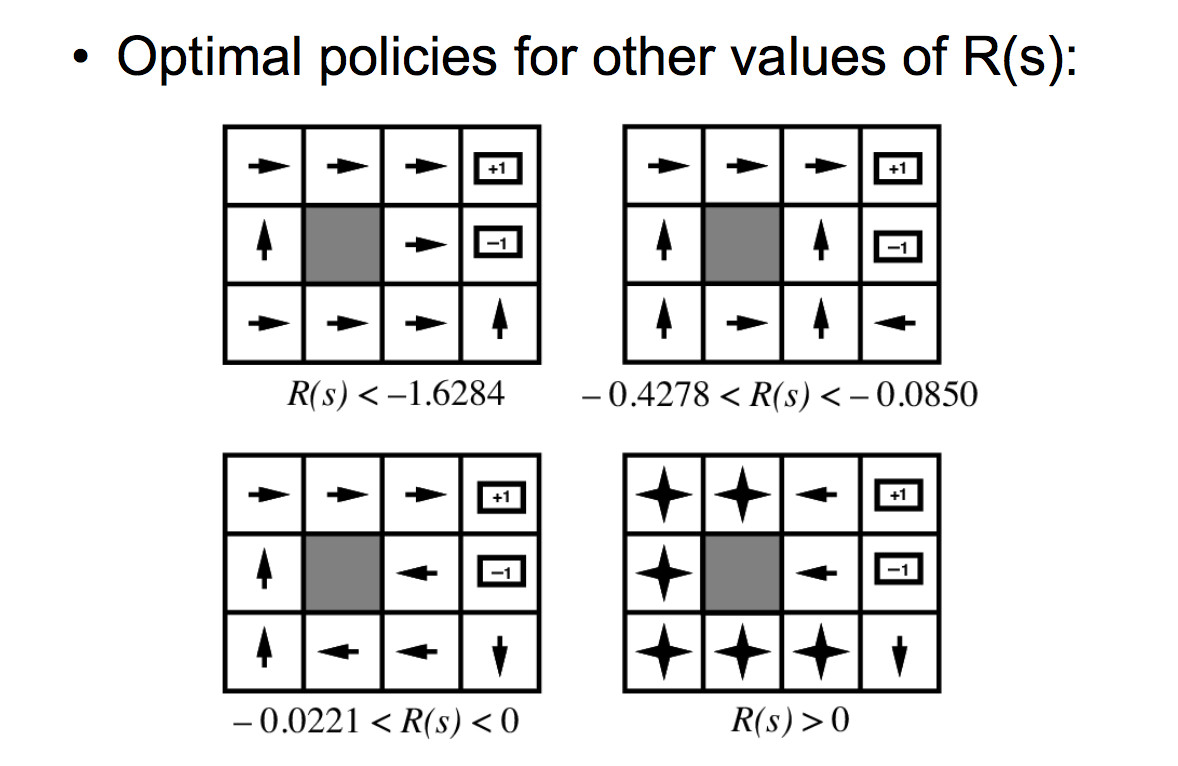CS 440/ECE 448
Margaret Fleck

## Reinforcement Learning 1

Gridworld pictures are from the U.C. Berkeley CS 188 materials (Pieter Abbeel and Dan Klein) except where noted.

## Reinforcement Learning

Reinforcement learning is a technique for deciding which action to take in an environment where your feedback is delayed and your knowledge of the environment is uncertain. Consider, for example, a driving exam. You excute a long series of maneuvers, with a stony-faced examiner sitting next to you. At the end (hopefully) he tells you that you've passed. Reinforcement Learning can be used on a variety of AI tasks, notably board games. But it is frequently associated with learning how to control mechanical systems. It is often one component in an AI system that also uses other techniques that we've seen.

For example, we can use reinforcement learning (plus neural networks) to learn how to park a car. The car starts off behaving randomly, but is rewarded whenever it parks the car, or comes close to doing so. Eventually it learns what's required to get this reward.

## Markov Decision Processes

The environment for reinforcement learning is typically modelled as a Markov Decision Process (MDP). The basic set-up is an agent (e.g. imagine a robot) moving around a world like the one shown below. Positions add or deduct points from your score. The Agent's goal is to accumulate the most points.It's best to imagine this as a game that goes on forever. Many real-world games are finite (e.g. a board game) or have actions that stop the game (e.g. robot falls down the stairs). We'll assume that reaching an end state will automatically reset you back to the start of a new game, so the process continues forever.

Actions are executed with errors. So if our agent commands a forward motion, there's some chance that they will instead move sideways. In real life, actions might not happen as commanded due to a combination of errors in the motion control and errors in our geometrical model of the environment.

## Mathematical setup

Eliminating the pretty details gives us a mathematician-friendly diagram:Our MDP's specification contains

• set of states $$s \in S$$
• set of actions $$a \in A$$
• reward function R(s)
• transition function P(s' | s,a)

The transition function tells us the probability that a commanded action a in a state s will cause a transition to state s'.

Our solution will be a policy $$\pi(s)$$ which specifies which action to command when we are in each state. For our small example, the arrows in the diagram below show the optimal policy.## The reward function

The reward function could have any pattern of negative and positive values. However, the intended pattern is

• A few states have big rewards or negative consequences.
• The rest of the states have some small background reward, often constant across all of these background states.

For our example above, the unmarked states have reward -0.04.

The background reward for the unmarked states changes the personality of the MDP. If the background reward is high (lower right below), the agent has no strong incentive to look for the high-reward states. If the background is strongly negative (upper left), the agent will head aggressively for the high-reward states, even at the risk of landing in the -1 location.## What makes a policy good?

We'd like our policy to maximize reward over time. So something like

$$\sum_\text{sequences of states}$$ P(sequence)R(sequence)

R(sequence) is the total reward for the sequence of states. P(sequence) is how often this sequence of states happens.

However, sequences of states might be extremely long or (for the mathematicians in the audience) infinitely long. Our agent needs to be able to learn and react in a reasonable amount of time. Worse, infinite sequences of values might not necessarily converge to finite values, even with the sequence probabilities taken into account.

So we make the assumption that rewards are better if they occur sooner. The equations in the next section will define a "utility" for each state that takes this into account. The utility of a state is based on its own reward and, also, on rewards that can be obtained from nearby states. That is, being near a big reward is almost as good as being at the big reward.

So what we're actually trying to maximize is

$$\sum_\text{sequences of states}$$ P(sequence)U(sequence)

U(sequence) is the sum of the utilities of the states in the sequence and P(sequence) is how often this sequence of states happens.